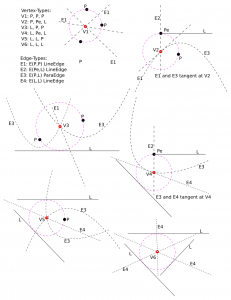## Towards medial-axis pocketing

Update: some work on connecting MICs together with bi-tangent line-segments, as well as a sequence of lead-out, rapid, lead-in, between successive MIC-slices: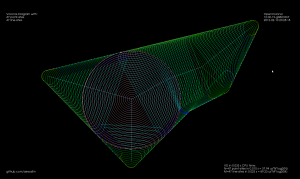It gets quite confusing with all cutting and non-cutting moves drawn in one image. The path starts with an Archimedean spiral (pink) that clears the initial largest MIC. We then proceed to "scoop" out the rest of the pocket with circular-arc cutting moves (green). At the end of a scoop we do a lead-out-arc (dark blue), followed by a linear rapid (cyan), and a lead-in-arc (light blue) to start the next scoop.

Next I'd like to put together an animation of this. The overall strategy will be much clearer from a movie.

This animation shows MICs, i.e. maximal inscribed circles (green, also called clearance-disks), inside a simple polygon (yellow). The largest MIC is shown in red.

This is work towards a new medial-axis pocketing strategy. The largest MIC (red) is first cleared using a spiral strategy. We then proceed to clear the rest of the pocket in the order that the MICs are drawn in the animation. We don't have to spin the tool around the whole circle. only the parts that need machining, which is the part of the new MIC that doesn't fall inside the previously machined MIC. As mentioned in my previous post this is inspired by Elber et al (2006).

The next step is to calculate how we should proceed from one MIC to the next, and how we do the rapid-traverse to re-position the tool for the next cut.

See also the spiral-toolpath by Held&Spielberger (2009) or their 199-slide presentation. The basics of this spiral-strategy is a straightforward march along the medial-axis. But then a filtering/fitting algorithm, which I don't have at hand right now, is applied to get the smoothed spiral-path.

## VD for polylines and polygons

I've been hacking away at openvoronoi, adding support for polylines and polygons.

The code I had in November works with individual non-intersecting line segments, like this: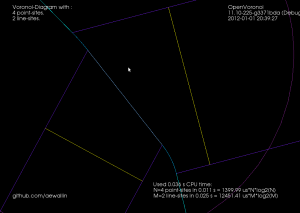Note how each vertex in the figure above is of degree three, i.e. there are three edges incident on each vertex. There's something about the number three, or triangles, or both, that makes planar graphs of degree three particularly nice to work with.

Here's the same figure with some notes describing the elements. The line-sites are drawn in yellow, and are associated with their left R(L+) and right R(L-) regions. The purple lines are called separators, and they define the region associated with the start- or end-point of a line-site. The three possible edge-types are also shown: LineEdge between two point-sites, LineEdge between two line-sites, and a Parabolic (PointLine) edge between a point-site and a line-site.Now, things get more complicated when we want to 'glue' two line-segments end-to-end in a polyline, or glue three or more line-segments together to form a polygon. Vertices are not necessarily of degree three any more. In fact the vertex degree is essentially unbounded, as you can start/end arbitrarily many line-segments at the same vertex. The solution I am using is to introduce what I call a null-face with zero-length null-edges around each point-site to which more than one line-segment connects. The mental picture is much like that of a key-chain, or mountaineering carabiners that are hooked-in to a loop. When we want to use the a vertex as a start/end-point for a segment we 'hook-in' to the null-face: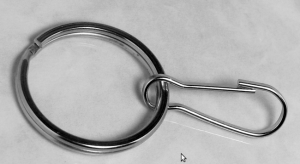This introduces a number of new rules and associated code for how vertices should be created, removed, and moved around a null-face, but it seems to work somehow now: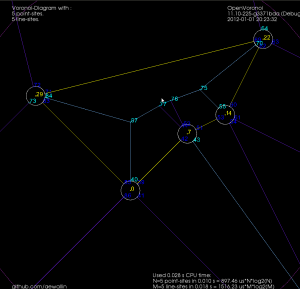Note how these null-faces and the circular null-edges around each end-point result in degree three vertices, which are much nicer to deal with in the algorithm. For example, the null-face around vertex "0" is 40->85->86->41->39. Without the null-face construction this vertex would be of degree five. Here is an annotated version of the same picture: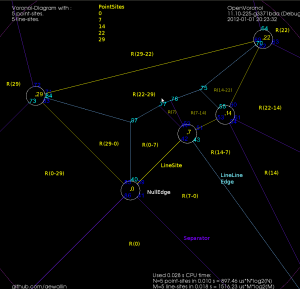This image shows how the diagram divides the plane into regions associated with endpoints such as R(0) and with the right/left side of a line-segment such as R(0-29) and R(29-0). The null-edges that form null-faces around each end-point are drawn in white.

Of course, these null-edges are only a topological construction. Geometrically we can position each vertex on a null-face at the location of the encircled point-site. This effectively contracts away the zero-area null-faces, and the result is the diagram we want: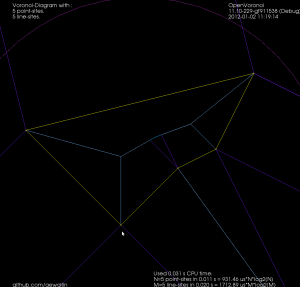The code now runs for a few select test-cases. To be continued...

Update: The code now seems to work also for random polygons with a large number of vertices. Here is one with 400 vertices: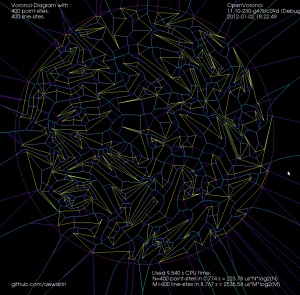and 3200-vertices: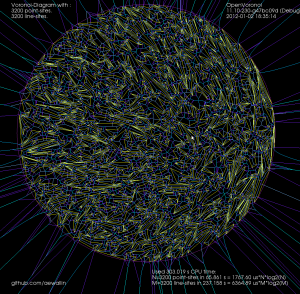## Poisson Voronoi diagram statistics

On g+, John Baez linked to work by Henk Hilhorst on the probability of finding voronoi cells with n sides in a poisson voronoi diagram.

A poisson voronoi diagram has point sites randomly and uniformly distributed, like this: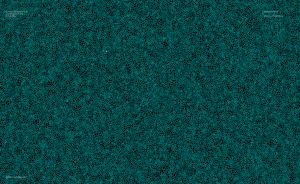Here's the distribution I came up with, after counting the edges for each voronoi cell from 200 runs where openvoronoi generated the diagram for 100k point-sites in each run. The green line is Hilhorst's prediction. I'm assuming poisson statistics, so if the bin-count for a certain n is fn, I've drawn errorbars of length sqrt(fn). Cells with n=15 or n=16 are so rare that the statistical error is of the same size as the probability (but it does agree with Hilhorst's prediction). Among the 20M cells I looked at I did not find n >= 17. For n=17 the predicted probability is 2e-9, so there's should be a 50% chance of seeing one if I would produce 500M random cells (that would take about 7 hours of CPU time).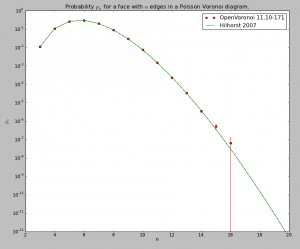The relevant papers are

The logarithmic y-axis hides the fact that the distribution is very peaked around n=6, so here is a plot with a linear y-axis: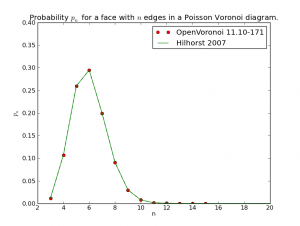## Random line-segment voronoi diagram

Update3: version 11.10-148 now goes to 16k line-sites without errors or warnings: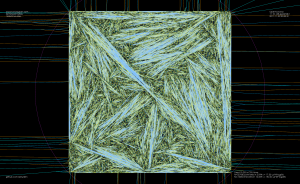Update2: This diagram with 8k vertices clearly has errors: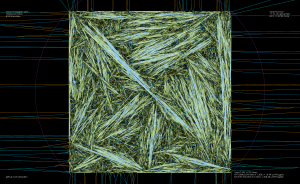Update: Version 11.10-141 now copes with 4k random segments. But I don't know of any smart way to check the diagram for correctness..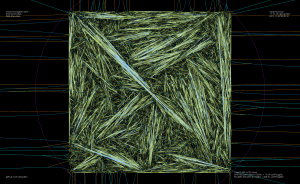Constructing the vd for random (non-crossing) line-segments is a reasonable stress-test for my vd-algorithm. When you've fixed one bug, just increase the number of line-segments by a factor of two and you will most likely uncover another! It now runs OK up to 2048 line-segments (yes, that does imply I get a segfault at 4096!).

There's some slowdown from 5us*n*log(n) in september 2011 (for just point-sites), to this code which runs in about 15 to 20us*n*log(n) when inserting the point-sites. Line-sites take longer, about 200us*m*log(m) for m line-sites.

## Voronoi diagram algorithm animations

I've made some more progress with my voronoi diagram code. I described the topology-oriented incremental algorithm earlier here (point sites) and here (line segments). When we want to add a new site (a point or a line-segment) to the diagram we should find a tree of to-be-deleted vertices/edges (red), create new (green) vertices on all in-out edges, and hook up the new vertices with new edges (green) to form the face for the new site. For point sites it looks like this(click thumbnail to see GIF animation!):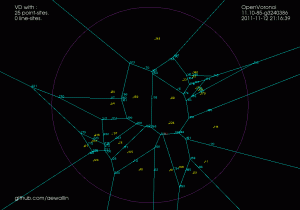For line-segment sites we need to modify the in_circle() predicate to calculate the minimum distance from a point to a line-segment. Then we need a much more involved solver that figures out points that are equidistant from points/lines. There are four different cases: point/point/point, point/point/line, point/line/line, and line/line/line (or you could identify a fifth and sixth type of vertex if you treat the case where one vertex is a line-segment endpoint differently, see drawing here).

In addition to simple line-edges between two point-sites we now also get two new types of edges: between line/line sites we have line-edges (but parametrized a little differently), and between point/line sites we have parabolic edges. The basic algorithm however looks pretty similar: we again find the delete-tree (red), create new vertices (green), and hook them up with new edges (green) to form a new face. (again click thumbnail to see GIF animation!).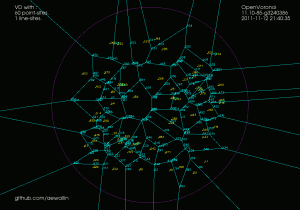There are (at least!) two more complications arising with line-sites that don't show up with just point-sites. These are handled with additional APEX and SPLIT vertices, which don't really add new geometry or topology to the diagram, they just make the algorithm handle certain special cases correctly.

It turns out that with parabolic edges there can be cases where both endpoints of the edge are marked IN (red, or to-be-deleted), but some parts of the edge still needs to be preserved. To handle this case correctly we insert a new type of APEX vertex at the apex of each parabolic edge. These APEX vertices can be seen at the tip of each parabola in the GIF-animation above.

The second special case is more subtle. There can be situations where the algorithm finds a to-be-deleted set of vertices that contains a loop/cycle. This is obviously forbidden because that would delete a face from the graph - which isn't allowed. That's why we require the delete-set to be a tree. Here is an image: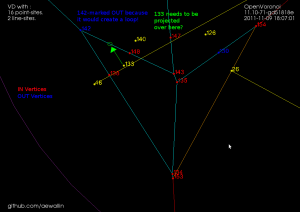We are inserting a new line-segment (yellow) starting at 48. The IN-vertices already found are colored red. Vertex 142 should be marked IN because it is closer to the new line-segment than to any other site. However that would create a loop of red vertices (134-135-143-148-142-138) and the face corresponding to the point-site 133 would be deleted. The problem is similar to that with APEX points: the end-points of edge 148-142 are both marked IN, but the edge should not be deleted completely. To handle this case we need to insert a SPLIT vertex at a position on the 148-142 edge which will be preserved(i.e. marked OUT). We do this by projecting the site 133 onto the edge 148-142 using the line-segment(yellow) as a mirror. This vertex will be marked OUT and ensures that the 148-142 edge is not completely removed. The same reasoning should work for circular arc-sites where we project radially through the center of the arc.

Here is a longer animation (best watched at 1080p/fullscreen!) which shows the insertion of 100 point-sites followed (after around 1:15) by insertion of (non-crossing!) line-segments:

The code now runs without errors until about 50 line-segments. There are problems with finding the proper end-points for separators in degenerate cases where the solver positions vertices on top of each other or very very close to each other.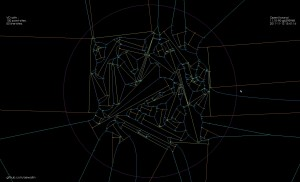Update: Some further bugfixes and filtering solution-points by both maximum and minimum t-value (clearance disk radius) has made the code run without assert()'s or problems up to N=100 line-segments: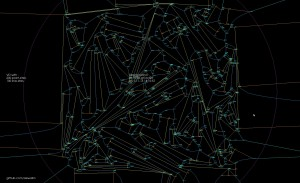Update2: now up to 200 line-sites: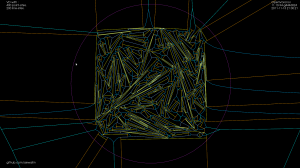Update3: Here is one case which I have identified as "hard" for the vd-vertex location solver code. We have as input to the solver a line-segment, one of its endpoints, and a third site (in this case also a line-segment). In some cases it happens that the offsets (dashed lines) of these three input sites are nearly parallel, which results in bad numerical precision, and the resulting solution can have an error which is as much as 1e9 times the normal errors I see.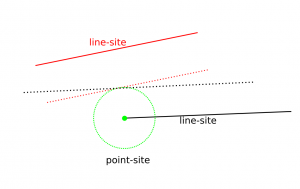## Line-segment voronoi diagram progress.

I've made some progress with my voronoi diagram code: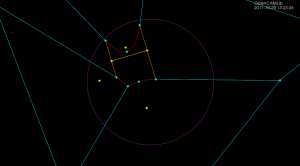Sites/generators in yellow. Line-edges in cyan, parabolic edges in red. Note that the parabolic edges are split at their apex, i.e. the closest point to the adjacent site.

Some problems with hooking up the half-edges of the two new faces that are associated with a new line-site remain. Hopefully soon to be fixed.

This code should in principle work for circular arc sites too. Adding arcs to the diagram results in elliptic and hyperbolic edges (in addition to line and parabola, which seem to work now).

## vd benchmark

Tuesday update: A few optimizations has shaved about half off the constant, to . This was mainly (a) choosing boost::vecS as the out-edge container for the BGL-graph, and (b) choosing std::vector instead of std::set for a temporary variable in the grid-search for the closest facet. I suspect the runtime is dominated by that grid-search. Profiling would tell.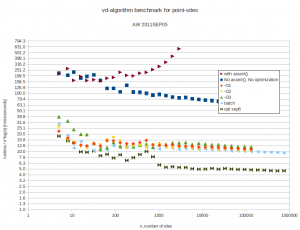I did some benchmarking of my vd-code. It is supposed to run in  time. Benchmarking turns out to be not so simple. I normally compile without any optimizations, and with a lot of sanity-checks and assert():s all over the code. These checks have worse time-complexity and result in long runtimes with big inputs. Then there are compiler optimization flags like -O or -O3.

I was first running the algorithm by inserting each point from python-code:

 t_before = time.time() for p in plist: vd.addVertexSite( p ) t_after = time.time() return (t_after - t_before)

which I thought could add some overhead. I also made a version ("batch mode") where we first push all points to c++, and then call a run() method:

 for p in plist: vd.pushVertexSite( p ) t_before = time.time() vd.run() t_after = time.time() return (t_after - t_before)

but this seems to improve runtime only marginally. Here are the results. Runtimes are divided by , so ideally all points should fall on a horizontal line. At best the code now runs in about 10-16 microseconds times .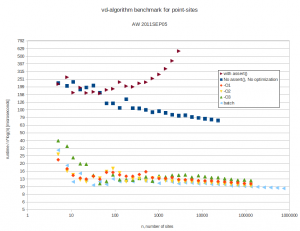With 4096 input points the runtime improves from 12s down to 1.3s by turning off sanity-checks and assert():s. It further improves to 0.18s with -O3 and to 0.165s in batch-mode.

As an example, the voronoi diagram for 1000 random points looks like this: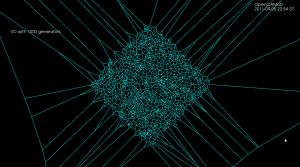## Line-segment voronoi diagram notes

The next step for the opencamlib voronoi-diagram (vd) algorithm is to be able to add line-segment generators. Some notes and ideas on that then (this turned into a rather long post...)

First let's recall (from February) how the point-generator vd-algorithm works: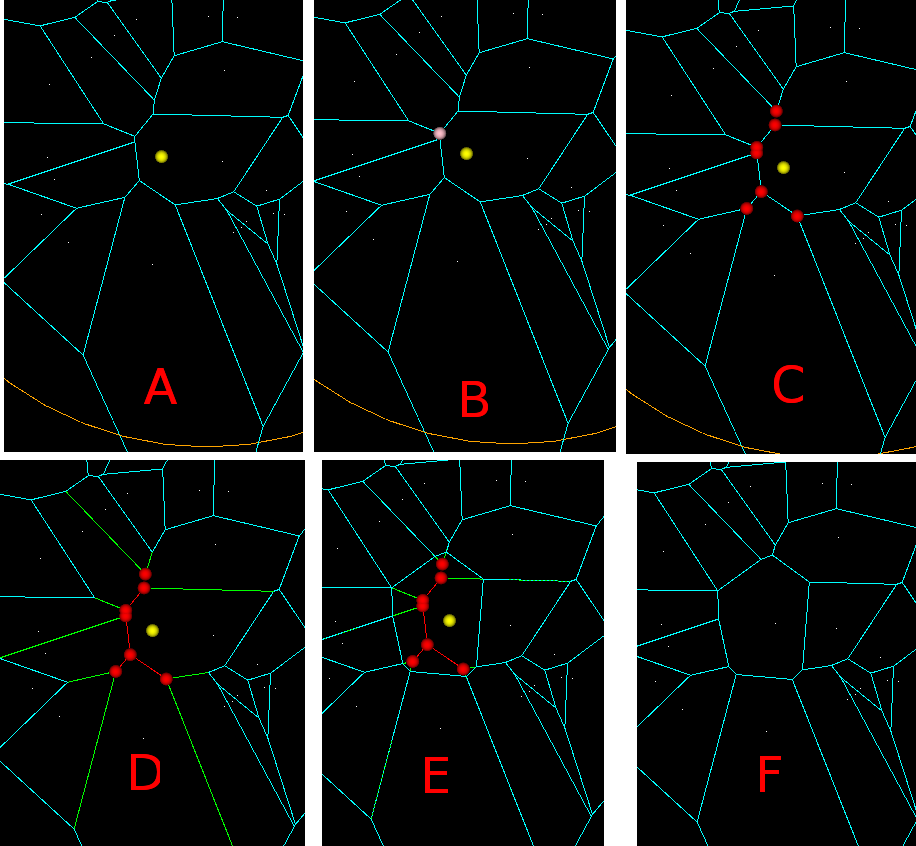In (A) we want to insert a new point-generator (yellow). First we need to identify the closest point-generator among the existing generators. (B) Then among the vertices that bound this voronoi-face(region) we search for a seed vertex (pink). The seed vertex is the one that violates the inCircle-predicate the most, i.e. has the minimum distance to the new point-to-be-inserted. (C) Starting from the seed vertex, we then identify other vertices (red) that need to be deleted, because they are closer to the new generator than to any other generator. These vertices are called "IN" vertices, and the associated edges (also in red) should (i) form a tree (a connected acyclic graph) and (ii) for any face, the "IN"-vertices should be connected, and the "OUT" vertices should be connected. (D) IN-IN edges (red) will be deleted completely, while NEW vertices will be generated on each IN-OUT edge (green). (E) NEW-NEW edges are created and connected into a cycle which forms the face/region for the new generator. (F) Now all IN-vertices and all IN-IN as well as IN-NEW edges are deleted and we are done.

Now the case when we insert a line segment. We start as above by constructing the diagram for all point-generators as well as all the endpoints of our line-segments. Here is an image from the Sugihara&Iri 2000 paper (http://dx.doi.org/10.1007/s004530010002) which I have enhanced with colors and text.The steps of the algorithm are similar to (A)-(F) above: In (A), since we have already inserted the two endpoints of the line-segment, we obviously know in which face to look for the seed-vertex. (B) when we search for a seed-vertex we now need both a dist(VDVertex, PointGenerator) function and a dist(VDVertex, LineGenerator) function. In (C) we again expand the set of "IN" vertices (red circles), while keeping the associated graph a tree, and not disconecting "IN"-vertices or "OUT"-vertices of any face (i and ii above) .

A new (iii) requirement is that the tree should connect the regions of the line-segment endpoints. A new situation also appears where the two endpoints of an edge are classified as "IN", but the complete edge should not be deleted. This is because edges between point and line-segment generators are parabolic arcs (curved!). In this case two NEW vertices are generated on the edge, so it splits into three parts: IN-NEW, NEW-NEW, and NEW-IN. This needs to be dealt with, but I'm not sure exactly how. I think one of the Held papers talks about avoiding this special case by splitting each parabolic edge in two, at the "vertex" of the parabola i.e. the point of greatest curvature (?).

"IN-IN" edges (magenta) will again be deleted. (D) NEW-vertices (green) should now be generated on each IN-OUT edge. This needs new functionality, since we need to deal with two types of edges: LineEdge and ParabolicEdge. The LineEdge occurs between two PointGenerators or between two LineGenerators, while the ParabolicEdge appears between a PointGenerator and a LineGenerator. The NEW vertex we need to generate will obviously be located on the IN-OUT edge (with associated generators gen1, gen2), and should be positioned so that it is equidistant from gen1, gen2, and the new line-segment generator. In contrast to the old point-generator diagram code where we only had one type of VDVertex, namely VDVertex( PointGenerator, PointGenerator, PointGenerator), we now have 6 (six!) different types of vertices in the diagram, depending on the three neighbouring generators, which can be of type LineGenerator, PointGenerator(endpoint), or PointGenerator:

• VDVertex( PointGenerator, PointGenerator, PointGenerator), type V1 in Okabe et al.
• VDVertex( PointGenerator, PointGenerator(endpoint) , LineGenerator), type V2
• VDVertex( LineGenerator, PointGenerator, PointGenerator), type V3
• VDVertex( LineGenerator, LineGenerator, PointGenerator(endpoint) ),  type V4
• VDVertex( LineGenerator, LineGenerator, PointGenerator ), type V5
• VDVertex( LineGenerator, LineGenerator, LineGenerator ), type V6

When these NEW-vertices (green) have been created, the next step is to connect them into a cycle with NEW-NEW edges (green). As we loop around the face we need to create either LineEdges or ParabolicEdges, depending on the neighbouring generators. Okabe lists the four different types of edges that appear in the diagram:

• VDEdge( PointGenerator, PointGenerator), type E1 (LineEdge)
• VDEdge( PointGenerator(endpoint), LineGenerator), type E2 (LineEdge)
• VDEdge( PointGenerator, LineGenerator), type E3 (ParabolicEdge)
• VDEdge( LineGenerator, LineGenerator), type E4 (LineEdge)

That's about it. It shouldn't be that hard to modify the current code to allow for six types of different VDVertex and four different types of VDEdge, and come up with a suitable design-pattern so that the correct type of vertex and edge are generated based on the type of the generators. Stay tuned...

Update1: here is another image with the same color-coding, from the Held VRONI-paper. The orange edge illustrates a case where the edge is marked "IN-IN" but in fact two new vertices should be generated on it, and the NEW-NEW portion of the edge is retained in the updated diagram (on the right).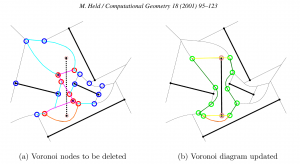Update2: Here is an image from the Held 2009 paper, which describes VDs for line-segment and circular arc generators. The circular arcs add a few additional special cases and some complexity, but the core of the algorithm remains the same: (1) find a seed vertex, (2) expand the set of 'marked' or "IN" vertices maximally, while keeping IN-IN edges (red) a tree, (3) generate NEW vertices (green) on IN-OUT edges (cyan), (4) hook up the NEW vertices with new edges (green) to form the new voronoi region, (5) delete IN-vertices, IN-IN edges, and IN-NEW edges.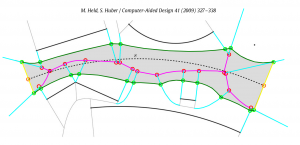Update3: Here's a drawing which shows the six different types of vertices.